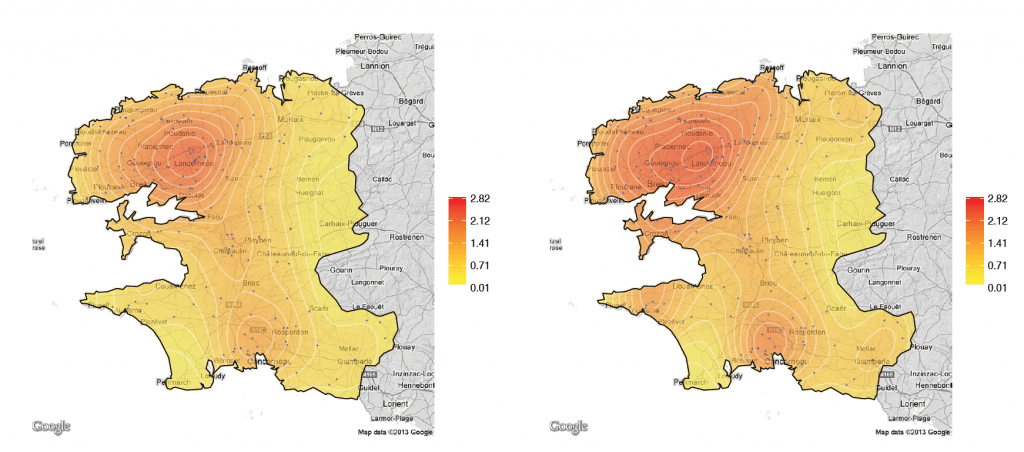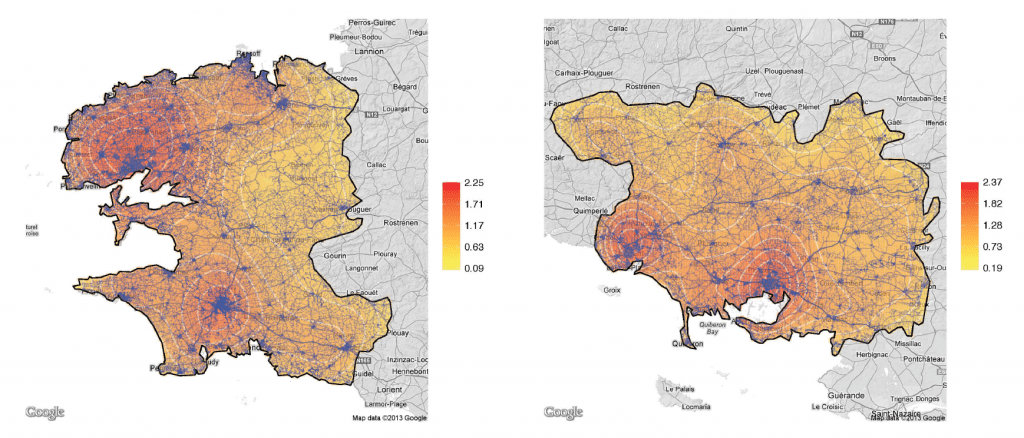# Visualizing densities of spatial processes

June 11, 2013
By

Want to share your content on R-bloggers? click here if you have a blog, or here if you don't.

We recently uploaded on http://hal.archives-ouvertes.fr/hal-00725090 a revised version of our work, with Ewen Gallic (a.k.a. @3wen) on Visualizing spatial processes using Ripley’s correction: an application to bodily-injury car accident location

In this paper, we investigate (and extend) Ripley’s circumference method to correct bias of density estimation of edges (or frontiers) of regions. The idea of the method was theoretical and di#cult to implement. We provide a simple technique – based of properties of Gaussian kernels – to compute e#efficiently weights to correct border bias on frontiers of the region of interest, with an automatic selection of an optimal radius for the method. An illustration on location of bodily-injury car accident (and hot spots) in the western part of France is discussed, where a lot of accident occur close to large cities, next to the sea.

Sketches of the R code can be found in the paper, to produce maps, an to describe the impact of our boundary correction. For instance, in Finistère, the distribution of car accident is the following (with a standard kernel on the left, and with correction on the right), with 186 claims (involving bodily injury)and in Morbihan with 180 claims, observed in a specific year (2008 as far as I remember),The code is the same as the one mentioned last year, except perhaps plotting functions. First, one needs to defi
ne a color scale and associated breaks

```breaks <- seq( min( result \$ZNA , na.rm = TRUE ) * 0.95 , max ( result\$ZNA , na.rm = TRUE ) * 1.05 , length = 21)
col <- rev( heat . colors (20) )```

to
finally plot the estimation

```image . plot ( result \$X, result \$Y, result \$ZNA , xlim = range (pol[,
1]) , ylim = range (pol[, 2]) , breaks = breaks , col = col ,
xlab = "", ylab = "", xaxt = "n", yaxt = "n", bty = "n",
zlim = range ( breaks ), horizontal = TRUE )```

It is possible to add a contour, the observations, and the border of the polygon

```contour ( result \$X, result \$Y, result \$ZNA , add = TRUE , col = "grey ")
points (X[, 1], X[, 2], pch = 19, cex = 0.2 , col = " dodger blue")
polygon (pol , lwd = 2)```

Now, if one wants to improve the aesthetics of the map, by adding a Google Maps base map, the
first thing to do – after loading ggmap package – is to get the base map

```theMap <- get_map( location =c( left =min (pol [ ,1]) , bottom =min (pol[ ,2]) , right =max (pol [ ,1]) ,
top =max (pol [ ,2])), source =" google ", messaging =F, color ="bw")```

Of course, data need to be put in the right format

```getMelt <- function ( smoothed ){
res <- melt ( smoothed \$ZNA)
res [ ,1] <- smoothed \$X[res [ ,1]]
res [ ,2] <- smoothed \$Y[res [ ,2]]
names (res) <- list ("X","Y","ZNA")
return (res )
}
smCont <- getMelt ( result )```

Breaks and labels should be prepared

```theLabels <- round (breaks ,2)
indLabels <- floor (seq (1, length ( theLabels ),length .out =5))
indLabels [ length ( indLabels )] <- length ( theLabels )
theLabels <- as. character ( theLabels [ indLabels ])
theLabels [ theLabels =="0"] <- " 0.00 "```

Now, the map can be built

```P <- ggmap ( theMap )
P <- P + geom _ point (aes(x=X, y=Y, col=ZNA), alpha =.3 , data =
smCont [!is.na( smCont \$ZNA ) ,], na.rm= TRUE )```

It is possible to add a contour

```P <- P + geom _ contour ( data = smCont [!is.na( smCont \$ZNA) ,] ,aes(x=
X, y=Y, z=ZNA ), alpha =0.5 , colour =" white ")```

and colors need to be updated

```P <- P + scale _ colour _ gradient ( name ="", low=" yellow ", high ="
red", breaks = breaks [ indLabels ], limits = range ( breaks ),
labels = theLabels )```

To remove the axis legends and labels, the theme should be updated

```P <- P + theme ( panel . grid . minor = element _ line ( colour =NA), panel
. grid . minor = element _ line ( colour =NA), panel . background =
element _ rect ( fill =NA , colour =NA), axis . text .x= element _ blank() ,
axis . text .y= element _ blank () , axis . ticks .x= element _ blank() ,
axis . ticks .y= element _ blank () , axis . title = element _ blank() , rect = element _ blank ())```

The
final step, in order to draw the border of the polygon

```polDF <- data . frame (pol)
colnames ( polDF ) <- list ("lon","lat")
(P <- P + geom _ polygon ( data =polDF , mapping =( aes(x=lon , y=lat)), colour =" black ", fill =NA))```

Then, we’ve applied that methodology to estimate the road network density in those two regions, in order to understand if high intensity means that it is a dangerous area, or if it simply because there is a lot of traffic (more traffic, more accident),We have been using the dataset obtained from the Geofabrik website which provides
Open-StreetMap data. Each observation is a section of a road, and contains a few points identifi
ed by their geographical coordinates that allow to draw lines. We have use those points to estimate a proxy of road intensity, with weight going from 10 (highways) to 1 (service roads).

```splitroad <- function ( listroad , h = 0.0025) {
pts = NULL
weights <- types . weights [ match ( unique ( listroad \$ type ), types .
weights \$ type ), " weight "]
for (i in 1:( length ( listroad ) - 1)) {
d = diag (as. matrix ( dist ( listroad [[i]]))[, 2: nrow ( listroad
[[i ]]) ]))
}}
return (pts )
}```

See Ewen’s blog for more details on the code, http://editerna.free.fr/blog/…. Note that Ewen did publish a poster of the paper (in French), for the http://r2013-lyon.sciencesconf.org/ conference, that will be organized in Lyon on June 27th-28th, see### Arthur Charpentier

Arthur Charpentier, professor in Montréal, in Actuarial Science. Former professor-assistant at ENSAE Paristech, associate professor at Ecole Polytechnique and assistant professor in Economics at Université de Rennes 1.  Graduated from ENSAE, Master in Mathematical Economics (Paris Dauphine), PhD in Mathematics (KU Leuven), and Fellow of the French Institute of Actuaries.

R-bloggers.com offers daily e-mail updates about R news and tutorials about learning R and many other topics. Click here if you're looking to post or find an R/data-science job.
Want to share your content on R-bloggers? click here if you have a blog, or here if you don't.